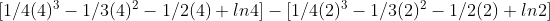# Modeling a Risk-Averse Investor in the Stock Market Pt. 3: Trying to Find a Closer Approximation for the net value

In this blogpost, I will attempt to find an approximation for the series posted in part 2. In part #2, we had the table:

Table 2. The table displays the X number of investments that are accruing value over an infinite J period. In our model, we had X = J hence setting J to infinity is an obvious over approximation. The shape produced by the cells is rectangular (J x X) where as in the true model it is triangular ~1/2(J x X).

Let’s change the definitions slightly and make the rectangular array into a square. Additionally, let’s also change the definition of each variable such that J = # of periods that accrue ROI and X = #investments + 1. For example, if we have 3 investments during 4 periods, then we have J = X where (J,X) is (4,4) array:

And so we can generalize the sum of each investment for all ROIs in the right hand column solutions as:

It isn’t clear to me how to mathematically dissect this series. One attempt is to represent it as an underapproximate in integral form:

Using our example case of Z = 4, this factors nicely by partial fractions to:

Where we can evaluate each individual giving us the indefinite form of:

We choose to use a Z = 4 as we have 3 investments for four periods of interest. Hence we need to calculate this through [4,2]. Expanding this out we have:(Eq 5) The integrated original integrated sum sums to roughly \$0.85

The above evaluation only works for Z = 4. If we had more ROI periods we could also have more investments and hence more terms to integrate. So we can try to generalize (#4).

We want to be able to write out the above integral type in a way that can be evaluated even when there’s 49 investments over 50 ROI periods. It wouldn’t make sense to hand write the 49 polynomials and 1 logarithm for the lower and upper bounds. So we can try to generalize the pattern for [2,Z] as:

So